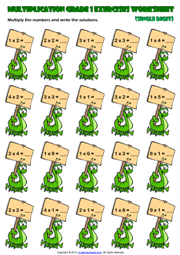Home Maths Main Page

# First Grade Single Digit Multiplication Exercise Worksheet

An easy multiplication exercise maths worksheet for grade 1 (first grade) students and kids with caterpillars theme. Multiply the numbers and write the solutions. Single Digit Multiplicants, Multipliers and results. (Horizontal Multiplication Worksheet for kids)Next Worksheet Grade 1 Multiplication Main Page Home